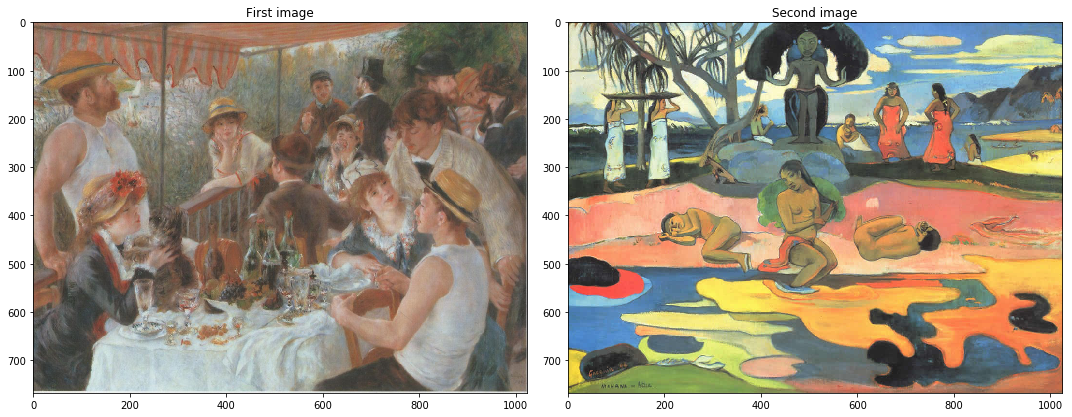# Introduction¶

For the following exercices, you need Python 3 with some basic librairies (see below). All images necessary for the session are available here.

If you use your own Python 3 install, you should download the images, put them in a convenient directory and update the path in the next cell.

For some parts of the session (cells with commands written as todo_something...), you are supposed to code by yourself.

In :
path = '../im/'

In :
import numpy as np
import matplotlib.pyplot as plt
import matplotlib
%matplotlib inline
from mpl_toolkits.mplot3d import Axes3D


The following line will be used to import the solutions of the practical session. Do not use it for the moment.

In :
from TP_color_transfer import *


Load and display a color image. A color image is made of three channels : red, green and blue. A color image in $\mathbb{R}^{N\times M}$ is stored as a $N\times M\times 3$ matrix.

Be careful with the functions plt.imread() and plt.imshow() of matplotlib.

• plt.imread() reads png images as numpy arrays of floating points between 0 and 1, but it reads jpg or bmp images as numpy arrays of 8 bit integers.

• In this practical session, we assume floating point images between 0 and 1, so if you use jpg or bmp images, you should normalize them to $[0,1]$.

• If 'im' is an image encoded as a double numpy array, plt.imshow(im) will display all values above 1 in white and all values below 0 in black. If the image 'im' is encoded on 8 bits though, plt.imshow(im) will display 0 in black and 255 in white.</span>

In :
imrgb1 = plt.imread(path+'renoir.jpg')/255
imrgb1=imrgb1[:,:,0:3] # useful if the image is a png with a transparency channel
imrgb2=imrgb2[:,:,0:3]

#we display the images
fig, axes = plt.subplots(nrows=1, ncols=2, figsize=(15, 7))
axes.imshow(imrgb1)
axes.set_title('First image')
axes.imshow(imrgb2)
axes.set_title('Second image')
fig.tight_layout()# Separable color transfer¶

## Separable affine transfer¶

Write a function affine_transfer which apply an affine transform to an image $u$ such that it has the same mean and the same standard deviation as $v$ on each channel.

In :
def affine_transfer(u,v):
w = np.zeros(u.shape)
for i in range(0,3):
w[:,:,i] = (u[:,:,i] -np.mean(u[:,:,i]))/np.std(u[:,:,i])*np.std(v[:,:,i])+ np.mean(v[:,:,i])
return w


Display and comment the result.

In :
w = affine_transfer(imrgb1,imrgb2)
w = (w>1)+(w<=1)*(w>0)*w      # w should be in [0,1]

In :
plt.figure(figsize=(7, 7))
plt.title('result of color separable affine transfer')
plt.imshow(w)

Out:
<matplotlib.image.AxesImage at 0x111770860>